## Solve the exercises Joint exercise page 83 (Chapter 9 Math 7 Connect) – Math Book

Solve the exercise Joint practice page 83 (Chapter 9 Math 7 Connect)
============

### Exercise 9.31, page 83, math 7, volume 2, problem solving skills

Prove that a triangle whose median and altitude from the same vertex coincide is an isosceles triangle.

Solution guide: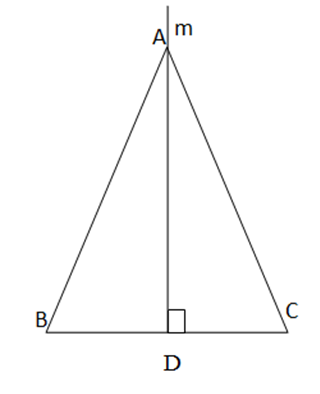From A draw a line m perpendicular to BC at midpoint D of BC

=> AD is the median of BC

DB = DC (D is mid point of BC)

=> AB= AC

=> ABC is balanced at A

### Exercise 9.32 page 83 math 7 volume 2 knowledge skills

Given three distinct points on the line A, B, C. Let d be the line perpendicular to AB at A. With the point M in d, M other than A, draw the line CM. Through B draw a line perpendicular to line CM, intersecting d at N. Show line BM, perpendicular to line CN

Solution guide: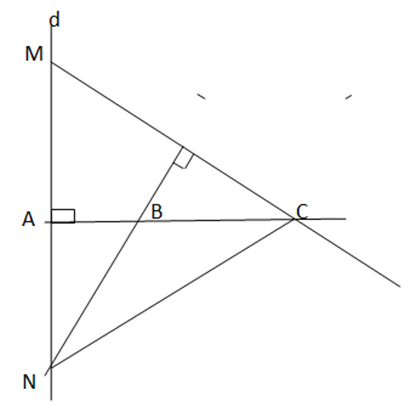We have: BN ⊥ CM, CA ⊥ MN. CA and BN cut each other in B

=> B is the orthocenter of MNC

=> MB CN

### Exercise 9.33 page 83 math 7 volume 2 knowledge skills

There is a round piece of corrugated iron that needs to be punched in the center. How to determine the center of that piece of corrugated iron?

Solution guide: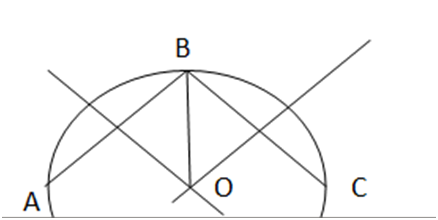• Take three distinct points A, B, C on the outer contour of the piece of corrugated iron.
• Draw the midline of side AB and side BC. These two orthogonal lines intersect at D. Then D is the center to be determined.

### Exercise 9.34 page 83 math 7 volume 2 knowledge skills

Let ABC be a triangle. Draw the bisector At of the angle formed by the ray AB and the opposite ray of AC. Prove that if the line containing the ray At is parallel to the line BC, then triangle ABC is isosceles at A.

Solution guide: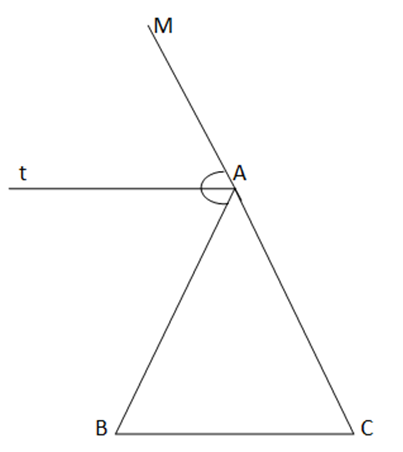Let AM be the opposite ray of AC. At is the bisector of $\widehat{MAB}$ => $\widehat{MAt}$ = $\widehat{tAB}$

We have At // BC => $\widehat{ABC}$ = $\widehat{tAB}$ ( 2 staggered angles)

$\widehat{ACB}$ = $\widehat{MAt}$ (2 isotopic angles)

where $\widehat{MAt}$ = $\widehat{tAB}$

=> $\widehat{ABC}$ =$\widehat{ACB}$

=> Triangle ABC is isosceles at A

### Exercise 9.35 page 83 math 7 volume 2 KNTT

The symbol S(ABC) is the area of ​​triangle ABC. Let G be the centroid of triangle ABC and M the midpoint of BC .

a) Show that S(GBC) = $\frac{1}{3}$ S(ABC)

Hint: use GM= $\frac{1}{3}$ AM to prove S(GMB) = $\frac{1}{3}$ S(ABM) , S(GCM) = $\frac{ 1}{3}$ S(ACM)

b) Prove that S(GCA) = S(GAB) = $\frac{1}{3}$ S(ABC)

Solution guide: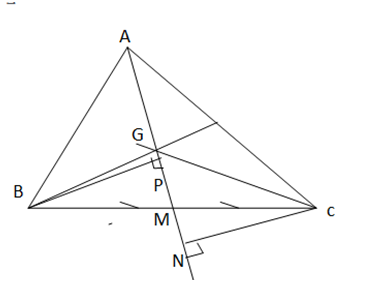a) Since G is the centroid of triangle ABC, GM= $\frac{1}{3}$ AM

BP ⊥ AM we have S (GMB)= $\frac{1}{2}$ BP . GM.

S ( ABM) = $\frac{1}{2}$ BP . AM.

We have S (GMB)= $\frac{1}{2}$ BP . GM.

=> S (GMB)= $\frac{1}{2}$ BP . $\frac{1}{3}$ AM

=> S (GMB) = $\frac{1}{3}$ AM. $\frac{1}{2}$ BP

=> S (GMB)= $\frac{1}{3}$ S (ABM) (1)

Similarly, draw CN ⊥ AM, we have S (GMC)= $\frac{1}{2}$ CN . GM.

S ( ACM) = $\frac{1}{2}$ CN . AM.

which GM= $\frac{1}{3}$ AM

=> S (GMC)= $\frac{1}{3}$ S (ACM) (2)

Adding both sides of (1) and (2) we have:

S (GMB) + S (GMC)= $\frac{1}{3}$ S (AMC) + $\frac{1}{3}$ S (ABM)

=> S( GBC) = $\frac{1}{3}$ S(ABC)

b) BP AM => BP AG

Sun AM => Sun AG

We have S (GAB)= $\frac{1}{2}$ BP . AG.

S (GAC)= $\frac{1}{2}$ CN . AG.

Considering ∆ square BPM at P and ∆ square CNM at N, there are:

BM=CM (M is mid point of BC)

$\widehat{PMB}$ = $\widehat{CMN}$ ( 2 opposite angles)

=> BPM = CNM

=> BP = CN

=> S (GAB) = S (GAC)

Yes AG= $\frac{2}{3}$ AM

S (ACB) = S (GAB) + S (GAC) + S ( GCB)

=> S (ACB) = S (GAB) + S (GAC) + $\frac{1}{3}$ S(ABC)

=> $\frac{2}{3}$ S( ABC) = 2 S (GAC)

=> $\frac{1}{3}$ S( ABC) = S (GAC) = S (GAB)